Practice the AP 10th Class Physical Science Bits with Answers Chapter 3 Refraction of Light at Plane Surfaces on a regular basis so that you can attempt exams with utmost confidence.

## AP State Syllabus 10th Class Physical Science Bits 3rd Lesson Refraction of Light at Plane Surfaces with Answers

CONCEPT – I : Refraction – Refractive index

Question 1.
The phenomenon of changing direction of light at interface of the two media when it travels from one medium to another obliquely is called ………………
A) Reflection
B) Refraction
C) Total Internal Reflection
D) Diffraction
B) Refraction

Question 2.
If v2 < v1 then medium 2 is said to be ……………… with respect ot medium 1.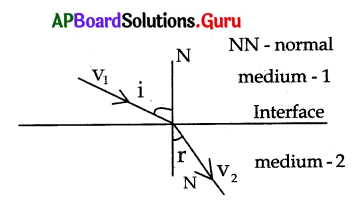A) denser
B) rarer
C) both
D) neither
A) denserQuestion 3.
The relation between the angle of incidence and the angle of refraction is ……………….. [from the above figure]
A) i = r
B) i > r
C) i < r D) i ≤ r Answer: B) i > r

Question 4.
During refraction …………….. at the interface of two media.
A) the speed of light does not change
B) the speed of light changes
C) frequency of light changes
D) All
B) the speed of light changes

Question 5.
Statement x: When light ray travels from a rarer medium to denser medium the refracted ray bends towards normal.
Statement y: When light ray travels from a rarer medium to denser medium the angle of refraction is less than the angle of incidence.
A) x and y are true
B) x and y are false
C) x is true, y is false
D) x is false, y is true
A) x and y are true

Question 6.
The extent of the change in direction that takes place when a light ray propagates through one medium to another medium is called ……………..
A) Refraction
B) Refractive index
C) Total internal reflection
D) None
B) Refractive index

Question 7.
The speed of light in vacuum is ……………………
A) 3, 00, 000 kms-1
B) 3 × 105 kms-1
C) 3 × 108 ms-1
D) all
D) all

Question 8.
The ratio of speed of light in vacuum to speed of light in that medium is called …………………
A) critical angle
B) refractive index
C) absolute refractive index
D) both B and C
D) both B and C

Question 9.
Statement x: Refractive index gives us an idea of how fast or how slow light travels in a medium.
Statement y: The speed .of light in a medium is low, when refractive index of medium is high.
A) x and y are true
B) x and y are false
C) x is true, y is false
D) x is false, y is true
A) x and y are trueQuestion 10.
Absolute refractive index n =
A) $$\frac{c}{\mathrm{v}}$$
B) $$\frac{v}{\mathrm{c}}$$
C) v × c
D) v ÷ c
A) $$\frac{c}{\mathrm{v}}$$

Question 11.
Match the following.

 Material medium Refractive index a) Air p) 1.44 b) Ice q) 1.33 c) Water r) 131 d) Kerosene s) 1. 0003

A) a → p,b → q,c → r,d → s
B) a → q,b → r,c → s,d → p
C) a → s,b → r,c → p,d → q
D) a → s,b → r,c → q,d → p
D) a → s,b → r,c → q,d → p

Question 12.
The refractive index of diamond is………………..
A) 2. 42
B) 24. 4
C) 1
D) 1. 42
A) 2. 42

Question 13.
Statement x: The refractive index of the kerosene is greater than the refractive index of water.
Statement y: An optically denser medium may not possess greater mass density.
A) both x and y are true
B) both x and y are false
C) x is true, y is false
D) x is false, y is true
A) both x and y are true

Question 14.
Which of the following is a dimension less quantity?
A) Refractive index
B) Relative refractive index
C) Both A and B
D) Speed of light
C) Both A and B

Question 15.
If the refractive index of glass is $$\frac{3}{2}$$ then the speed of light in glass is
A) 3 × 108 ms-1
B) 2 × 108 ms-1
C) $$\frac{9}{2}$$ × 108 ms-1
D) $$\frac{3}{2}$$
B) 2 × 108 ms-1

Question 16.
Refractive index of a medium depends on ………………..
A) nature of material
B) wavelength of light
C) both A and B
D) neither A nor B
C) both A and BQuestion 17.
When light is going from one medium (other than vacuum or air) to another medium then the value of refractive index is called ……………..
A) absolute refractive index
B) relative refractive index
C) both A and B
D) neither A nor B
B) relative refractive index

Question 18.
The ratio of speed of light in first medium to speed of light in second medium is called …………………..
A) absolute refractive index of first medium
B) refractive index of 2nd medium with respect to 1st medium
C) refractive index of 1st medium with respect to 2nd medium
D) all of the above
B) refractive index of 2nd medium with respect to 1st medium

Question 19.
Relative refractive index n21 = ………………
A) $$\frac{n_{2}}{n_{1}}=\frac{v_{1}}{v_{2}}$$
B) $$\frac{\mathrm{n}_{2}}{\mathrm{n}_{1}}=\frac{\mathrm{v}_{2}}{\mathrm{v}_{1}}$$\frac{n_{1}}{n_{2}}=\frac{v_{1}}{v_{2}}
C) $$\frac{n_{1}}{n_{2}}=\frac{v_{1}}{v_{2}}$$
D) $$\frac{\mathrm{c}}{\mathrm{v}}$$
A) $$\frac{n_{2}}{n_{1}}=\frac{v_{1}}{v_{2}}$$

Question 20.
Which of the following is Snell’s law ?
A) n1 sin i = sin r/n2
B) n1/n2 = sin r/sin i
C) n2/n1 = sin r/sin i
D) n2 sin i = constant
B) n1/n2 = sin r/sin i

Question 21.
Refractive index of glass relative to water is 9/8. The refractive index of water relative to glass is …………………
A) 8
B) 1.33
C) 1.5
D) 8/9
D) 8/9

Question 22.
During refraction light follows ………………………..
A) Snell’s law
B) Archimedes law
C) Pascal’s law
D) Galilio’s law
A) Snell’s law

Question 23.
Which of the following effect is not related to refraction ?
A) A coin kept at a bottom of vessel filled with water appears to be raised
B) A lemon kept in a glass of water appears to bigger than its size
C) A thick glass slab is placed over some printed letters, they appear raised when viewed through glass slab.
A) A coin kept at a bottom of vessel filled with water appears to be raised

Question 24.
Which of the following effect is not related to refraction ?
A) A pencil held obliquely and partly immersed in water appears to be bent at the water surface.
B) A pool of water appears to be less deep than it actually is.
C) The images formed by spherical mirrors.
D) The stars appear to twinkle on a clear night.
C) The images formed by spherical mirrors.Question 25.
Which of the following diagram shows the ray of light refracted correctly ?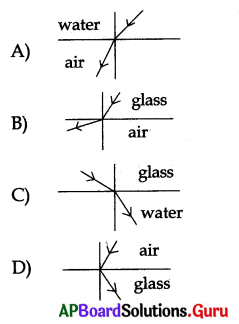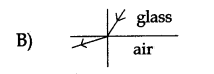Question 26.
The refractive indices of four substances P, Q, R and S are 1.50,1.36,1.77 and 1.31 respectively. The speed of light is the minimum in the substance.
A) S
B) R
C) Q
D) P
B) R

Question 27.
The refractive indices of four materials P, Q, R and S are 1.33,1.31,1.44 and 1.52 respectively. When the light rays pass from air into these materials, they refract the maximum in …………………
A) P
B) Q
C) R
D) S
D) S

Question 28.
The refractive index of glass for light going from aif’to glass is $$\frac{3}{2}$$. The refractive index of air for light going from glass to air ………………….
A) 1
B) $$\frac{3}{2}$$
C) $$\frac{4}{6}$$
D) $$\frac{5}{2}$$
C) $$\frac{4}{6}$$

Question 29.
The refractive index of glass with respect to air is $$\frac{3}{2}$$ and the refractive
index of water with respect to air is $$\frac{3}{2}$$ . The refractive index of glass with
respect to-water will be
A) $$\frac{3}{2}$$
B) $$\frac{3}{4}$$
C) $$\frac{9}{8}$$
D) $$\frac{8}{9}$$
C) $$\frac{9}{8}$$

Question 30.
A light ray incident on curved surface travelling from rarer medium to denser medium bends ………………….. the normal.
A) towards
B) away from
C) parallel to
D) none
A) towards

Question 31.
If i and r be the angles of incidence and refraction respectively, when the light ray travels from glass to air, then
A) i = r
B) i >r
C) i < r
D) none
C) i < rQuestion 32.
If the refractive index of transparent material is $$\frac{3}{2}$$the speed of light in that
medium (in ip/s) is ……………………..
A) 4.5 × 108
B) 4.5 × 1010
C) 2 × 108
D) 2 × 1010
C) 2 × 108

Question 33.
When light enters from air to a medium “X” its speed becomes 2 Answer: 108 ms-1, then refractive index of the medium ‘X’ is …………………
A) 2
B) 3
C) 1.5
D) 1
C) 1.5

Question 34.
If n is the refractive index of a medium and v is the velocity of light in that medium, then which one of the following statements is correct ?
A) If n is low, v is low
B) If n is high, v is low
C) n = v for all media
D) n and v are independent of each other
B) If n is high, v is low

Question 35.
The angle of refraction of a light ray is the angle between …………………
A) incident ray and refracted ray
B) refracted ray and interface separating the two media
C) normal ray and incident ray
D) normal ray and refracted ray
D) normal ray and refracted ray

Question 36.
The extent of the change in the direction that takes place when a light ray travels from one medium to another is given by………………..
A) critical angle
B) focal length
C) refractive index
D) focal power
C) refractive index

Question 37.
The refractive index of a material is 1. If the speed of light in vacuum is 3 × 108 ms-1 then the speed of light in that material is ………………..
A) 0.3 × 108 m/s
B) 3 × 108 m/s
C) 4 × 108 m/s
D) 108 m/s
B) 3 × 108 m/s

Question 38.
Speed of light is almost half of the vacuum in which of the following ?
A) Air
B) Water
C) Glass
D) Kerosene
C) GlassQuestion 39.
Kerosene is optically ………………than water
A) rarer
B) denser
C) dilute
D) higher
B) denser

Question 40.
In which of the following case a light ray does not deviate from its path at the interface of two media ?
A) It is incident normally
B) Refractive indices of two media is same
C) It is incident obliquely
D) Both A and B
D) Both A and B

Question 41.
A diamond shines more than a glass piece cut to the same shape due to………..
a) refractive index of the diamond is high than the glass piece
b) critical angle of diamond is low than the glass piece
c) critical angle of diamond is high than the glass piece
d) refractive index of the diamond is low than the glass piece
A) a and b
B) c and d
C) a and c
D) b and d
A) a and b

Question 42.
Ramu poured some glycerine into the glass vessel and then poured water up to the brim. He kept the quartz glass red into the vessel while doing an activity. When he see the glass rod from the sides of the glass vessel, he can see the ………………….
A) only submerged part of the glass rod into the glycerine
B) only submerged part of the glass rod into the water
C) total length of the glass rod into the vessel
D) none
B) only submerged part of the glass rod into the water

Question 43.
nair = 1, c = 30° (glass – air) the n for glass is ………………………
A) 0
B) 1
C) 2
D) 3
C) 2

Question 44.
Speed of light in a medium is ………………. than in vacuum.
A) less
B) greater
C) equal
D) none
A) less

Question 45.
Speed of light in vacuum is “c” and n = 3/2 then the speed of light in medium is ………………….
A) $$\frac{3 c}{2}$$
B) $$\frac{2 c}{3}$$
C) $$\frac{5 c}{7}$$
D) $$\frac{7 c}{5}$$
B) $$\frac{2 c}{3}$$

Question 46.
Refractive index of Benzene is …………………….
A) 1.53
B) 1.50
C) 1.47
D) 1.63
B) 1.50Question 47.
Find the refractive index of the medium from the figure.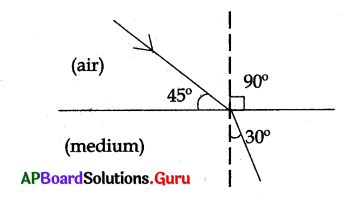A) 2
B) 1.414
C) $$\frac{1}{2 \sqrt{2}}$$
D) $$\frac{1}{\sqrt{2}}$$
B) 1.414

CONCEPT – II : Total Internal Reflection

Question 48.
When the angle of incidence is greater than the critical angle the light ray is ! reflected into denser medium at inter face. This phenomenon is called ………………….
A) critical angle
B) reflection
C) refraction
D) total internal reflection
D) total internal reflection

Question 49.
At critical angle of incidence the angle of refraction is ……………………..
A) 0°
B) 90°
C) 45°
D) 180°
B) 90°

Question 50.
Total internal reflection takes place when the light ray travels from ………………………..
A) rarer to denser medium
B) rarer to rarer medium
C) denser to rarer medium
D) denser to denser medium
C) denser to rarer medium

Question 51.
The angle of incidence at which the light ray travels from denser to rarer medium grazes the interface is called ……………………….
A) refracted angle
B) reflected angle
C) critical angle
D) supplementary angle
C) critical angle

Question 52.
Which of the following conditions are needed to take “Total Internal Reflection”?
a) Light rays travel front denser to rarer medium
b) Light rays travel fromrarer to denser medium
c) Angle of incidence is greater than critical angle
d) Angle of incidence is smaller than < critical angle
A) only (a)
B) (a) and (c)
C) (b) and (d)
B) (a) and (c)

Question 53.
Statement x : Refractive index of air increases with height.
Statement y : Cooler air has greater refractive index than , hotter air.
A) x is true, y is false
B) x is false, y is true
C) Both are true
D) Both are false
C) Both are trueQuestion 54.
Statement x : If the temperature of air increases, the refractive index of air decreases.
Statement y : The refractive index of air does not depend on its temperature
A) Both are true
B) Both are false
C) x is true, y is false
D) x is false, y is true
A) Both are true

Question 55.
The value of refractive index of a rock salt is ………………
A) 2. 52
B) 1.54
C) 2. 62
D) 1.42
B) 1.54

Question 56.
The critical angle of a diamond is ……………..
A) 24.4°
B) 42.4°
C) 14.4°
D) 2.42°
A) 24.4°

Question 57.
Which one of the following is not an application of total internal reflection ?
A) Shining of diamond
B) Optical fibre
C) Mirage
D) Blue colour of sky
D) Blue colour of sky

Question 58.
In what condition ray grazes along die surface?
A) ∠i > ∠c
B) ∠i = ∠c
C) ∠i < ∠c
D) ∠i = 0
B) ∠i = ∠c

Question 59.
A ball coated with lamp black put in a glass tank containing water appears shining due to …………………
A) refraction
B) diffraction
C) interference
D) total internal reflection
D) total internal reflection

Question 60.
The refractive index and the critical angle of a medium are n and c respectively then
A) n = c
B) n = sin c
C) n = $$\frac{1}{\sin c}$$
D) n = $$\frac{1}{\mathrm{c}}$$
C) n = $$\frac{1}{\sin c}$$Question 61.
The absolute refractive index of water is. $$\frac{4}{3}$$. The critical angle of water is
A) sinc = $$\frac{4}{3}$$
B) c = sin-1( $$\frac{4}{3}$$)
C) c = sin-1 ($$\frac{3}{4}$$)
D) 0°
C) c = sin-1 ($$\frac{3}{4}$$)

Question 62.
The absolute refractive index of a medium is 2. The critical angle for that medium ……………….
A) sin-1 = ($$\frac{1}{3}$$)
B) sin-1 (2/3)
C) sin-1($$\frac{1}{2}$$)
D) sin-1(3/2)
C) sin-1($$\frac{1}{2}$$)

Question 63.
Statement X: If angle of incidence is less than critical angle refraction takes place.
Statement Y: If angle of incidence is greater than critical angle refraction does not take place. (AS 2)
A) Both statements are true
B) Both statements are false
C) Statement – X is true, statement – Y is false
D) Statement – X is false, statement – Y is true
A) Both statements are true

Question 64.
As temperature of medium increases, the critical angle ………………..
A) increases
B) decreases
C) remains same
D) first increases then decreases
A) increases

Question 65.
The refractive index of a material is 2, then the critical angle is ……………………..
A) sin-1(2)
B) sin-1($$\frac{1}{2}$$)
C) sin-1(√2)
D) sin-1($$\frac{1}{\sqrt{2}}$$)
B) sin-1($$\frac{1}{2}$$)

Question 66.
The total internal reflection in a diamond makes it shine, because the critical angle of diamond is ……………….
A) very low
B) very high
C) exactly 45°
D) none
A) very low

Question 67.
The main principle behind working of optical fibres is …………………..
A) total internal reflection
B) dispersion
C) refraction
D) reflection
A) total internal reflectionQuestion 68.
Choose the correct increasing order of substances water, ice, kerosene arranged based on their refractive index ………………….
A) ice, water, kerosene
B) ice, kerosene, water
C) water, ice, kerosene
D) water, kerosene, ice
A) ice, water, kerosene

Question 69.
If n1 and n2 be the refractive Indices of denser ancfrarer media respectively and 1 c is the critical angle, then ………………..
A) sin c = $$\frac{n_{1}}{n_{2}}$$
B) sin c = $$\frac{n_{2}}{n_{1}}$$
C) sin c = $$\sqrt{\mathrm{n}_{2} / \mathrm{n}_{1}}$$
D) sin c = $$\sqrt{\mathrm{n}_{1} / \mathrm{n}_{2}}$$
B) sin c = $$\frac{n_{2}}{n_{1}}$$

Question 70.
A bunch of optical fibres causes a ……………………..
A) mirage
B) light pipe
C) cable
D) telescope
B) light pipe

Question 71.
Refractive index of medium is directly proportional to ………………..
A) mass density of the medium
B) optical density of the medium
C) frequency of the light ray
D) all the above
B) optical density of the medium

Question 72.
To see internal organs of the human body optical fibres are used in ……………….
A) physiotherapy
C) endoscopy
D) microscopy
C) endoscopy

Question 73.
If critical angle of the medium is 60°, then the velocity of that medium is …………………..m/s
A) $$\frac{3}{2}$$ × 108
B) $$\frac{3 \sqrt{3}}{2}$$ × 108
C) $$\frac{2}{3}$$ × 108
D) 2√3 × 108
A) $$\frac{3}{2}$$ × 108

Question 74.
Relation between critical angles of water and glass is ………………..
A) cw < cg
B) cw = cg
C) cw > cg
D) Done
C) cw > cgCONCEPT – III : Refraction Through A Glass slab

Question 75.
The light ray that falls perpendicular to one side of the glass slab surface comes out……………………..
A) with deviation
B) without any deviation
C) both A and B
D) can’t say
B) without any deviation

Question 76.
When a glass slab is introduced in the path of a light ray the number of times the light ray undergoes refraction are ……………….
A) 2 times
B) 3 times
C) 4 times
D) 1 time
A) 2 times

Question 77.
Refractive index of the glass slab =
A) $$\frac{\text { Thickness of the glass slab }}{\text { Vertical shift }}$$
B) $$\frac{\text { Thickness of the glass slab }}{\text { Lateral shift }}$$
C) $$\frac{\text { Thickness of the glass slab }}{\text { Thickness of the glass slab – verticalshift }}$$
D) $$\frac{\text { Thickness of the glass slab }}{\text { Thickness of the slab – lateral shift }}$$
C) $$\frac{\text { Thickness of the glass slab }}{\text { Thickness of the glass slab – verticalshift }}$$

Question 78.
The lateral displacement depends on ………………….
A) angle of incidence
B) thickness of the slab
C) both A and B
D) none
C) both A and B

Question 79.
The angle between emergent ray and normal is called ………………
A) angle of incidence
B) angle of refraction
C) angle of emergence
D) normal
C) angle of emergence

Question 80.
The perpendicular distance between the extended emergent ray and incident ray which are parallel is called
A) lateral shift
B) lateral image
C) object distance
D) none
A) lateral shift

Question 81.
The lateral displacement between the incident and the emergent rays ………………… when the angle of incidence increases.
A) increases
B) decreases
C) remains same
D) does not depend on angle of incidence
A) lateral shift
A) increasesQuestion 82.
The lateral displacement between the incident and the emergent rays …………….., when the thickness of the glass slab increases
A) increases
B) decreases.
C) remains-same
D) none
A) increases

Question 83.
The angle of deviation produced by the glass slab
A) 0°
B) 20°
C) 90°
D) depends on the angle formed by the light ray and normal to the slab
A) 0°

Question 84.
Thickness of a glass slab is 3 cm and its refractive index is 2, then its vertical
shift is…………………
A) 1.5
B) 3.5
C) 2.5
D) 2
A) 1.5

Question 85.
Thickness of a glass slab is 3 cm and vertical shift is 1.5, then its refractive index is ……………………..
A) 3
B) 2
C) 1.5
D) 2.5
B) 2

Question 86.
The net deviation produced by a rectangular glass slab is …………………..
A) equal to angle of incidence
B) greater than angle of incidence
C) less than angle of incidence
D) zero always
D) zero always

Question 87.
Raju is doing an experiment with the glass slab. He focussed the light towards the glass slab at an angle 30°. What would be tire angle of emergence?
A) 30°
B) 0°
C) 90°
D) >30°
A) 30°Question 88.
In the diagram, identify the angles which are correctly marked ?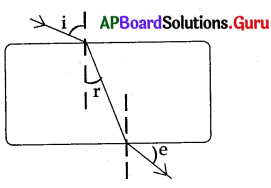A) ∠i and ∠r
B) ∠r and ∠e
C) ∠r and ∠e
D) ∠i, ∠r and ∠e
A) ∠i and ∠r

Question 89.
Which of the following is correct from Hie above diagram ?
A) ∠i > ∠r
B) ∠r < ∠i
C) ∠i = ∠e
D) all
D) all

Question 90.
If the incident ray falls normally on the surface of a glass slab then trace out the correct diagram ?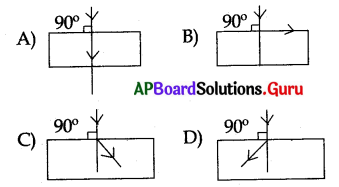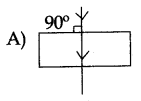Question 91
A ray of light falls normally on a glass slab. What is the angle of refraction ?
A) 0°
B) 90°
C) 45°
D) 180°
A) 0°

Question 92.
A ray of light falls normally on a glass slab. What is the angle of emergence ?
A) 0°
B) 90°
C) 45°
D) 180°
A) 0°

Question 93.
A ray of light falls obliquely on a glass slab. What is the angle of emergence ?
A) Greater than incident angle
B) Less than incident angle
C) Equal to incident angle
D) 0°
C) Equal to incident angle

Question 94.
When a ray of light travels through glass slab. Which of the following is not true when it is incidentobliquely ?
A) Light ray bends towards the normal when it travels from air to glass.
B) Light ray bends away from the normal when it travels from glass to air
C) Incident ray and emergent ray lie along the same straight line.
D) Incident ray and emergent ray are not along the same straight line.
D) Incident ray and emergent ray are not along the same straight line.Question 95.
Ramu placed a rectangular glass slab over printed letters. The letters appears while ………………. viewing through a glass slab.
A) away from its original position
B) nearer as its original position
C) as its original position
D) both A and B
B) nearer as its original position

Question 96.
If the thickness’ of the glass slab increases then the refractive index of the glass slab …………………
A) decreases
B) increases
C) remains same
D) none
C) remains same

Question 97.
If the vertical shiff produced by the glass slab increases then the refractive index of the glass slab ………………………
A) decreases
B) increases
C) remains same
D) none
C) remains same

Question 98.
When the object is in denser medium’ and the observer is in rarer medium, the object should appear …………………..
A) closer than the original position
B) away from its original position
C) as its original position
D) both A and B